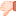Indexing Question Please Help! -- Stuck

Consider the relation R(A,B,C,D), consisting of 100,000 tuples. Each page contains 10 tuple entries. R is saved as a heap with dense secondary indexes on B. Data is distributed randomly. The attribute B lies densely in [1, 100,000] i.e., each value in [0, 100,000] occurs in B. Consider these three methods of access:
A1.reading the entire heap file for R (no index)
A2.access by means of a B+ Tree on B.
A3.access by means of a hash-index on B
(extensible hash, directory in main memory)

(i) Assume that the application uses mainly point queries regarding attribute B. Estimate the minimal and the maximal number of block accesses that each of the methods A1, A2, and A3 need for point queries. Explain your answers. Which method requires the smallest number of accesses for the minimal case and which for the maximal case?

(ii) Assume that the application uses mainly range queries. Estimate the minimal and the maximal number of block accesses that each of the methods A1, A2, and A3 need for range queries. Explain your answers. Which method requires the smallest number of accesses for the minimal case and which for the maximal case?